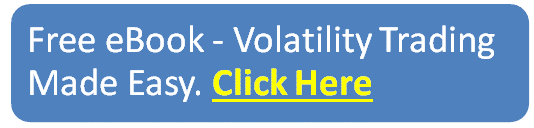blog

# The Ultimate Guide To Call Ratio Spreads

#### Options Trading 101 - The Ultimate Beginners Guide To Options

As Seen On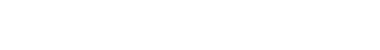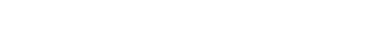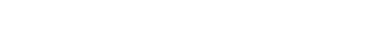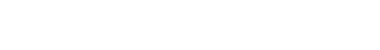by Gavin in Blog
November 3, 2020 1 comment## Guide to Call Ratio Spreads

A call ratio spread is an option trade that involves buying call options and selling a different number of call options on the same instrument and the same expiration date.

The name of the trade comes from the ratio between the number of long and short positions.

There are two types of call ratio spreads, the first one is the call front ratio spread or simply call ratio spread, and the second one is the call ratio backspread.

In this article, we are going to discuss the call front ratio spread. Here’s what it looks like just so you know you’re in the right place.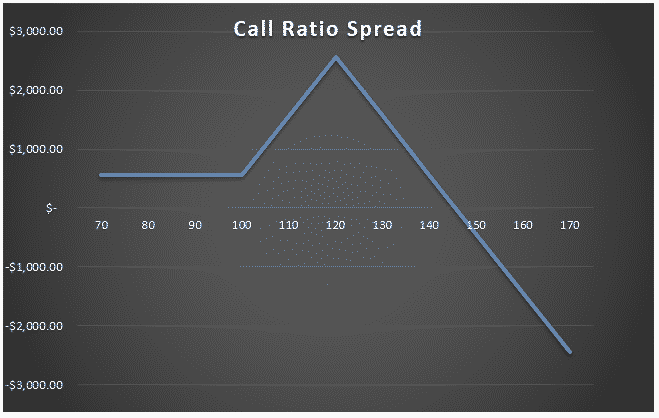The most common ratio in call ratio spreads is two-to-one, where there are twice as many short calls as long calls.

The strategy is profitable when the stock price slightly rises or the implied volatility falls.

A typical call ratio spread is created buying one at-the-money (ATM) call option and selling two call options at a higher strike price (OTM).

There is a unlimited risk if the stock rallies and it moves above the short strike of the call options.

Some investors use call ratio spreads when they expect that the stock will not move too much and want to profit on the downside.

Call ratio spreads are considered more conservative strategies than selling naked calls and the trade has usually higher breakeven price.

The margin cost associated with the naked call piece of a call ratio spread is high and the investor would need the highest option approval level to sell naked calls.

Here is an example of a SPY call ratio spread.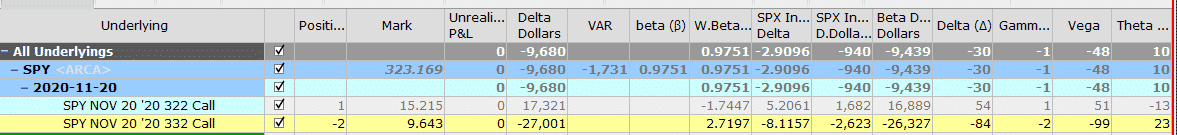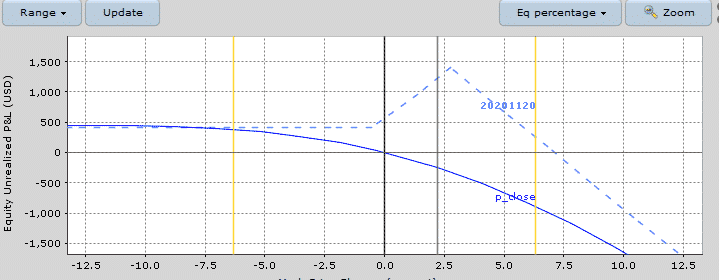## Maximum Loss

If the stock rallies above the strike price of the short call options, the maximum loss is unlimited.

In this SPY case, there is no loss on the downside because the trade was created for a credit.

In the SPY example above, the strikes are \$10 apart, one long SPY 322 call, and two short SPY 332 calls.  The trade loses money when SPY ends above \$346.

## Maximum Gain

The maximum gain occurs when the underlying is at the price of the short calls at expiration. In this ideal situation, the short calls would expire worthless and the long call would be in-the-money.

Maximum Gain = difference between the strike prices + credit received when the position was initiated (or debit)

In our SPY example, as the strikes are \$10 wide, the maximum gain is \$1000 + \$407 = \$1407.

## Breakeven Price

If the call ratio spread is established for a credit, there is only one breakeven price which is:

Upper breakeven price = strike price of the short calls + difference between the strikes + net credit received.

In our SPY example, the breakeven price is \$332 + \$10 + \$4.07 = \$346.07

If the call ratio spread is established for a debit, there are two breakeven points:

Lower breakeven price = strike price of the long call + Net debit paid

Upper breakeven point = strike price of the short calls + difference between the strikes – net debit paid

## Payoff Diagram

Call ratio spreads have a slanted tent-shaped payoff diagram. This kind of shape results in high gamma near expiry.

If the trade is created for a credit, call ratio spreads have no risk on the downside, and a tent-shaped profit zone on the upside.

The downside risk starts as soon as the stock price overpasses the breakeven price on the right. However, losses can occur before the stock reaches the short strike if the stock makes a quick move.

For this reason, a big rally in the stock is the worst-case scenario.

Here you can see on the T+0 line that losses start to occur as soon as the stock rallies.

Below you ca find the payoff graph from OptionNet Explorer showing the T+0, T+19 and T+38 lines. This should give you a good appreciation of how the trade progresses over time.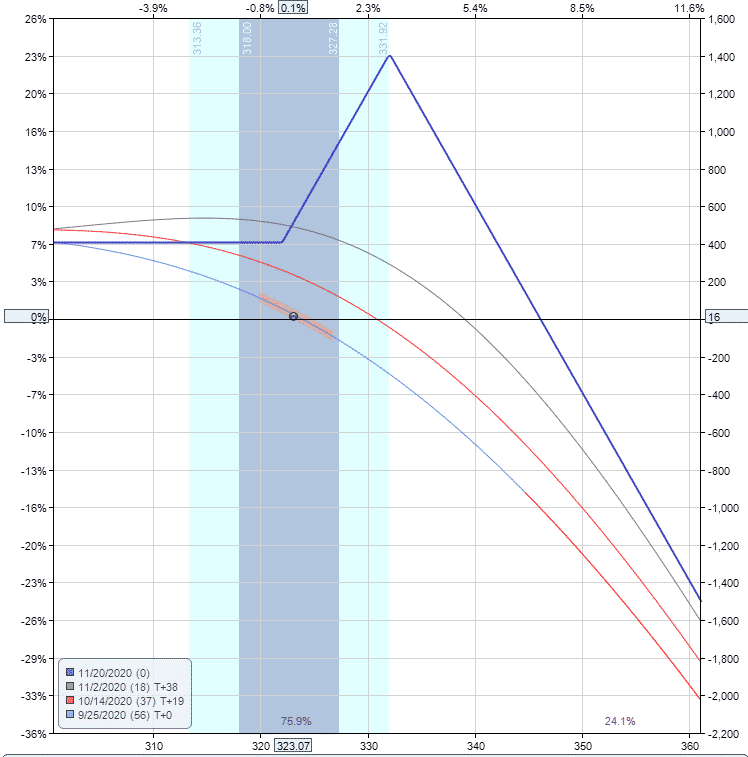## Risk of Early Assignment

There is always a risk of early assignment if the stock rallies above the short call strike price.

You can mitigate this risk by trading Index options, but they are more expensive.

Usually, an early assignment only occurs on call options when there is an upcoming dividend payment. Traders will exercise the call to take ownership of the share before the ex-date and receive the dividend.

For this reason, it is important to watch out for ex-dividend dates.

Otherwise, make sure to close the trade before the short calls go in-the-money and this will help avoid early assignment.

## How Volatility Impacts Call Ratio Spreads

Call ratio spreads are short vega trades, so they benefit from falling volatility after the trade has been placed.

Our SPY example starts with a vega of -48. This means that for every 1% drop in implied volatility, the trade should gain \$48.

The opposite is true if implied volatility rises 1% – the position would lose \$48.

Here you can see the potential benefit a 10% drop in implied volatility could have. Chang in volatility can have a big impact on this trade.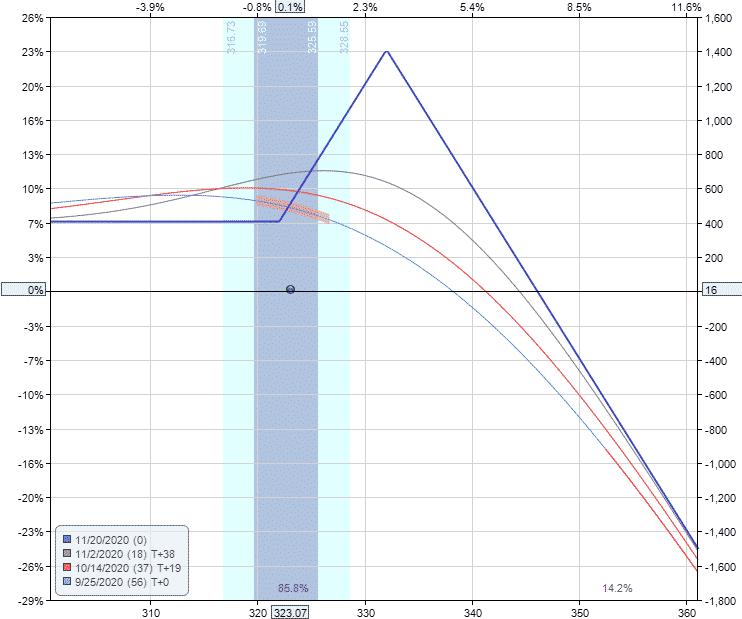## How Theta Impacts Call Ratio Spreads

Call ratio spreads are positive theta trades, they make money as time passes, with all else being equal.

This is because the short calls suffer faster time decay than the long call.

In our SPY example, the trade has positive theta of 10. This means that all else being equal, the trade will gain \$10 per day due to time decay.

Notice that the positive time decay on the short-term sold call (23) is higher than the time decay being suffered on the longer-dated long call (-13).

## Other Greeks

### Delta

Delta on a call ratio spread can be positive or negative, depending on how far out-of-the-money are short calls.

Traders who prefer to create the position for a net credit will have negative delta call ratio spreads at the beginning of the trade.

Positive delta call ratio spreads are usually created for a net debit.

As the trade progresses, the delta will change.

### Gamma

Call ratio spreads are negative gamma, they will benefit from stable prices.

In other words, you want the stock to stay relatively flat with a moderate increase in price to finish close to the short call strike.

In our SPY example, the initial call ratio spread had -1 gamma. Gamma will have the least impact out of all the greeks.

## Risks

The main risk with the trade is a sharp rally in the underlying stock breaking through the short call strike.

### Assignment Risk

Assignment risk is highest if the stock is trading ex-dividend and the short call is in the money.

One way to avoid assignment risk is to trade stocks that do not pay dividends or trade indexes that are European style and cannot be exercised early. The risk is also very low if the short calls are out-of-the-money.

To reduce assignment risk consider closing your trade if the short calls are close to being in-the-money, particularly if it is close to expiry.

### Expiration Risk

Leading into expiration, if the stock is trading above the shorts call, the trader has expiration risk.

The most important risk is that the trader might get assigned and there was not enough money in the account to cover the position.

A margin call would be triggered in this case.

The best way to avoid this type of risk is to simply close out the position, or at least the extra call in the spread before expiry.

### Volatility Risk

We saw earlier that implied volatility can have a big impact  on this type of option trade, so traders need to have a good understanding of this.

The combined vega of the short calls will generally be much greater than the long call.

The volatility of the position changes with the duration of the trade.

## Short Call vs Call Ratio Spread

There are some obvious differences between short calls and call ratio spreads.

Call ratio spreads can be seen as a combination of a bull call spread and a naked call.

The presence of the bull call spread makes the time-decay of the trade slower than the theta we have in a naked call trade.

Both trades have unlimited risk on the upside, but call ratio spreads have a higher breakeven point than the naked short trade.

Looking at our SPY call ratio spread we have that the breakeven point was \$346.07.

Simply selling the 332 call would result in a breakeven price of \$332 + \$9.64 = \$341.64.

Call ratio spreads have a higher maximum gain than naked short calls.

Our SPY call ratio spread had a maximum gain of \$1407 against \$964 we would get from a naked call 332 SPY.

As with all trading strategies, it is important to plan how you are going to manage the trade in any scenario.

What will you do if the stock rallies? What about if it drops? Where will you take profits? Where and how will you adjust? When will you get stopped out?

Let us discuss some of the basics of how to manage call ratio spreads.

### Profit Target

It is important to have a profit target. That might be 10% of the margin used in the trade or you may plan on holding to expiration provided the stock stays below the short strike calls.

For call ratio spreads, 10-15 percent is a good profit target.

### Stop Loss

Having a stop loss is also important, perhaps more so than the profit target.

With a call ratio spread, you can set a stop loss based on a percentage of the margin required.

In this case, it might be closing the trade if the loss reaches 15-20% following a rally in the stock.

One easy adjustment if the stock rallies early in the trade is to delta hedge. This allows the trade to temporarily neutralize the price risk while letting time decay do it’s thing.

## Example

Based on the assumption that stock TWTR will not rally in the next 45 days,  we create a call ratio spread buying the ATM September 25 38.50 call and we sell two 41.50 calls with the same expiry.

The breakeven point of the trade is 41.5 + 3 – 0.03 = 44.47.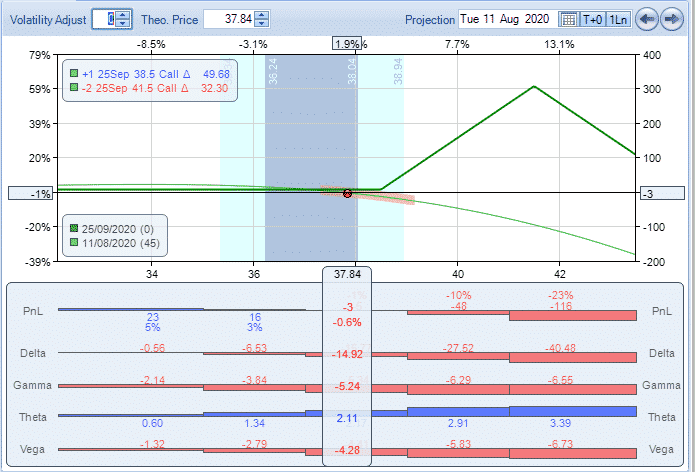As time goes, theta erodes the price of the short calls and the trade makes money.

After one month in the trade, we made \$60 from a maximum gain of \$300 (spread) -\$3 = \$297, a profit of 20%.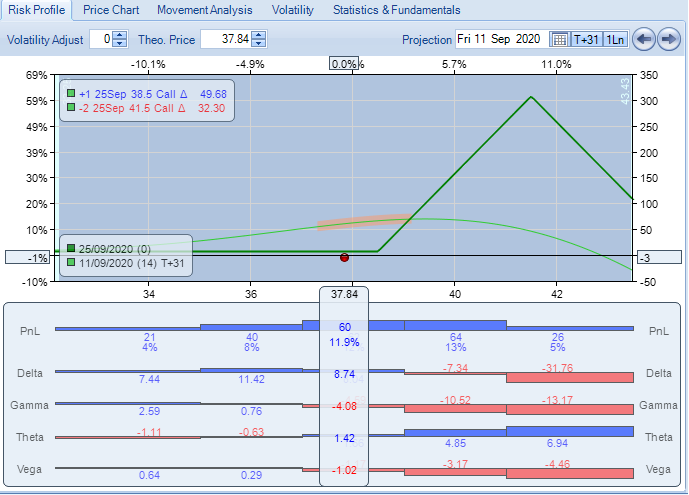## Summary

Call ratio spreads are an option strategy to consider when you expect the stock will not rally and you want some generate some income.

For this reason, they should only be placed on stocks the trader thinks might move slightly higher but not too much higher.

A good time to enter this type of trade is at the end of a strong bull move where further upside is likely to be limited.

One nice feature of the trade is that there is very little risk on the downside and in some cases, even the ability to generate a small amount of premium if the stock drops.